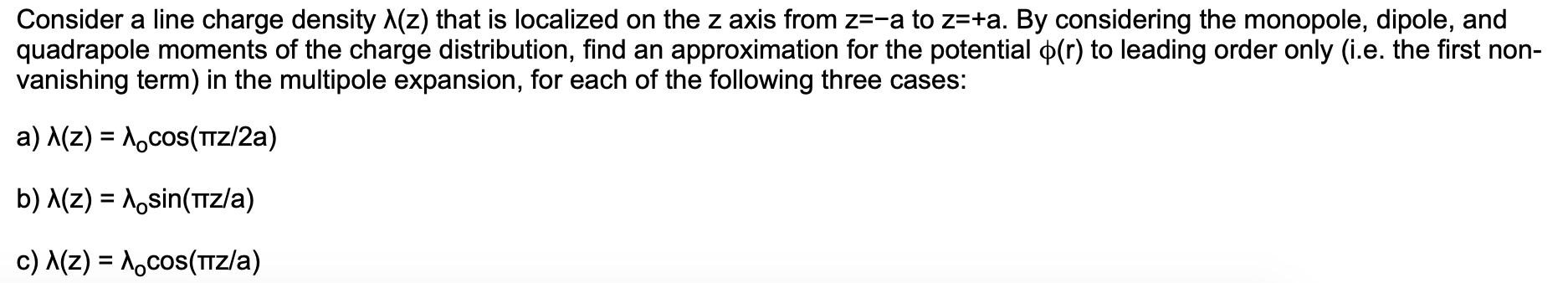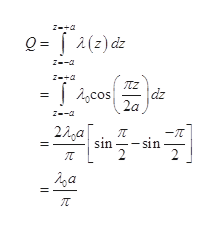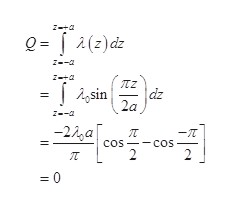# Consider a line charge density A(z) that is localized on the z axis from z=-a to z=+a. By considering the monopole, dipole, andquadrapole moments of the charge distribution, find an approximation for the potential p(r) to leading order only (i.e. the first non-vanishing term) in the multipole expansion, for each of the following three cases:a) A(z) Acos(TZ/2a)b) A(z) Aosin(TZ/a)c) MZ) Aocos(Tz/a)

Question
9 views

It's an electromagnetics problem.help_outlineImage TranscriptioncloseConsider a line charge density A(z) that is localized on the z axis from z=-a to z=+a. By considering the monopole, dipole, and quadrapole moments of the charge distribution, find an approximation for the potential p(r) to leading order only (i.e. the first non- vanishing term) in the multipole expansion, for each of the following three cases: a) A(z) Acos(TZ/2a) b) A(z) Aosin(TZ/a) c) MZ) Aocos(Tz/a) fullscreen
check_circle

star
star
star
star
star
1 Rating
Step 1

(a)

Write the expression for the monopole momenthelp_outlineImage Transcriptionclosez-+a Q= 2(2) dz z--a z-+a = j 4,cos| dz 2a 22ga -T - sin sin 2 fullscreen
Step 2

Substitute the monopole moment in the multipole expansion

Step 3

(b)

Write the expression for t...help_outlineImage TranscriptioncloseZ-+a Q= 2(z)dz 22)dz z--a dz A,sin 2a -22a -T - cos 2 cos 0 fullscreen

### Want to see the full answer?

See Solution

#### Want to see this answer and more?

Solutions are written by subject experts who are available 24/7. Questions are typically answered within 1 hour.*

See Solution
*Response times may vary by subject and question.
Tagged in

### Science